Courses
Courses for Kids
Free study material
Free LIVE classes
More

# Displacement, Velocity and Acceleration Time Graphs for JEELIVE
Join Vedantu’s FREE Mastercalss

## An Introduction to Motion

Motion, as the name suggests, refers to the change of position with change in time. To study any kind of motion, various terms are defined in physics, some of which we’ll be discussing in this article. Let’s start with displacement. Displacement refers to the shortest distance between the initial and final position of any object. Unlike distance, displacement is a vector quantity. The difference between distance and displacement can be understood by taking the following example.

Suppose Riya runs a 500m race around a circular track. Then, the distance travelled by Riya will be 500 metres. But, the displacement would be zero, as the final and initial position would be the same for a circular track, and therefore the shortest distance between final and initial position would become zero.

Velocity is defined as the displacement per unit time. It can be positive, negative or zero as it is a vector quantity.  The zero velocity refers to no change in the position, or simply we can say that the body is at rest.

Acceleration is defined as the rate of change of velocity. When the acceleration is positive, it means that the velocity is increasing with time. A negative acceleration indicates that the velocity is decreasing with time. And if the acceleration is zero, then it means that the velocity is constant.

In this article, we’ll study displacement time graph velocity time graph acceleration time graph.

## Displacement-Time Graph

The displacement time graph or time displacement graph is used to study the variation of displacement with time and to find the velocity of the object in motion. The time is represented at the horizontal axis and displacement at the vertical axis. Below are certain characteristics of the graph.

1. If the motion is uniform, then the graph obtained is a straight line with a constant slope. This means that the velocity is constant.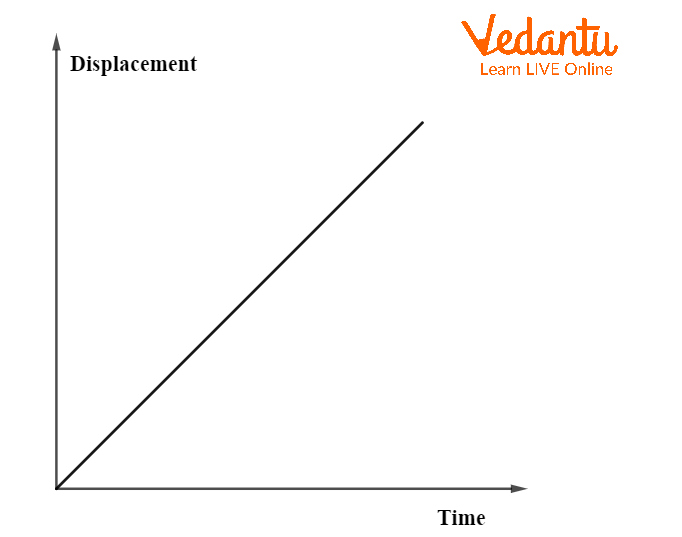Displacement v/s Time Graph for Uniform Motion

1. If there is no motion in the body, then a straight horizontal line is obtained in the graph. This indicates that the velocity is zero.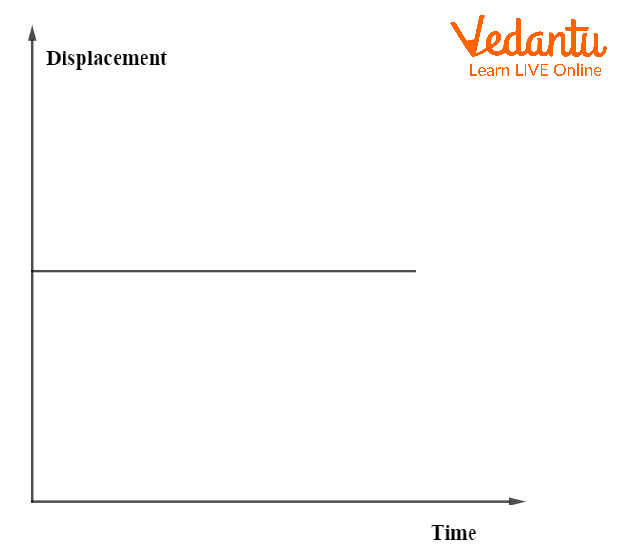Displacement v/s Time Graph When the Body is at Rest

1. If there is a uniform acceleration, then a parabola is obtained in the graph. This refers to a change in velocity with time that can be understood with the concept of acceleration.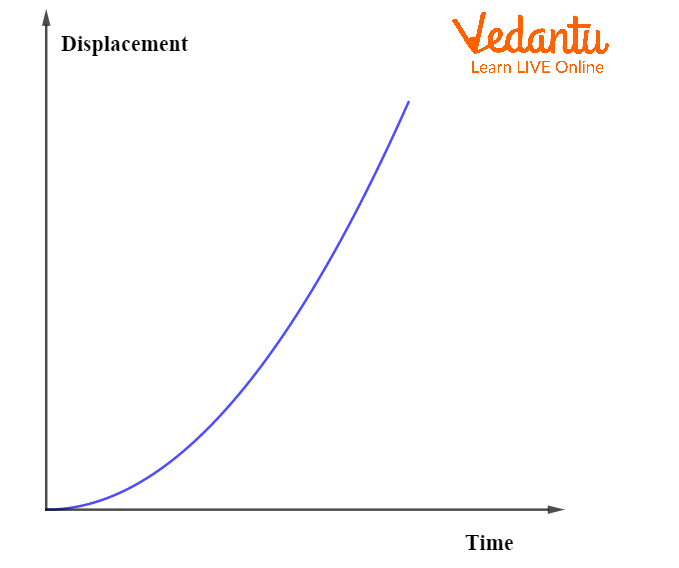Displacement-time Graph for Uniform Acceleration

The slope of a displacement time graph gives the velocity of the object. It can be determined by dividing the change in displacement by change in time as shown below. Consider the following graph.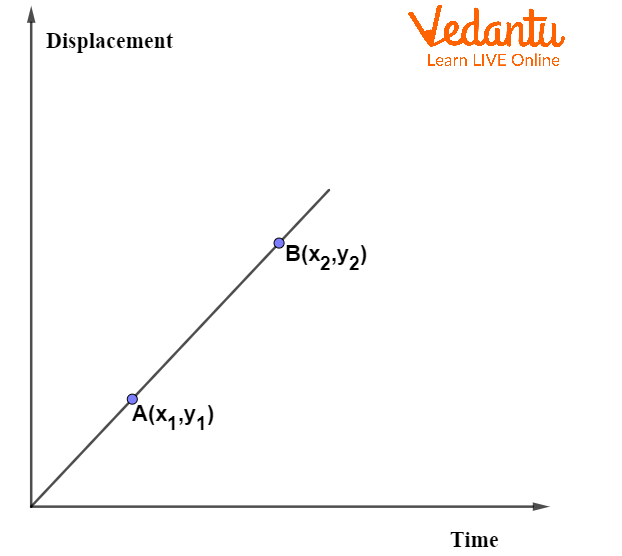Linear Straight Line Displacement v/s Time Graph

In the above graph, the change in displacement (s) between points A and B is shown.

The change in time (t) between points A and B is $t={{x}_{2}}-{{x}_{1}}$

Then,  the slope of the graph will be $\dfrac{s}{t}=\dfrac{{{y}_{2}}-{{y}_{1}}}{{{x}_{2}}-{{x}_{1}}}$ . This is also the velocity of the object.

## Velocity-Time Graph Displacement

The velocity-time graph is used to study the variation of velocity with time and to find the acceleration. The time is represented at the horizontal axis and velocity at the vertical axis. There are certain characteristics of the graph.

1. When the velocity is constant, a straight line parallel to the horizontal axis is obtained. This refers to zero acceleration.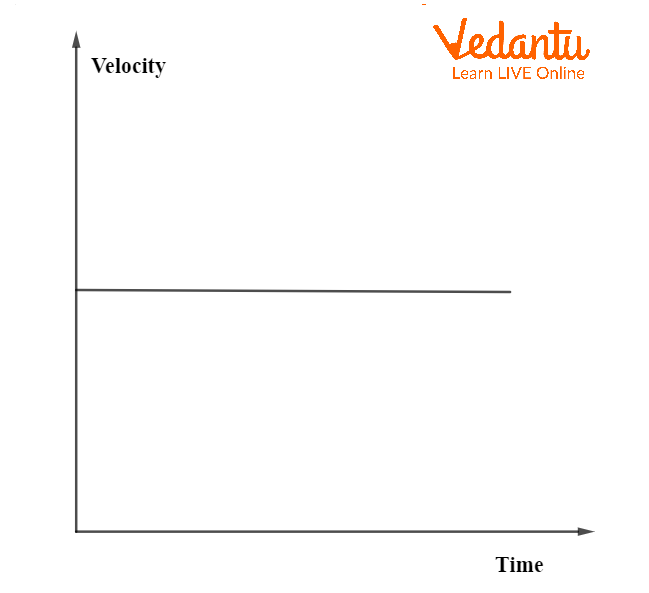Velocity v/s Time Graph for Constant Velocity

1. When the acceleration is uniform and the velocity is constantly increasing, the graph obtained is a straight line with a positive slope.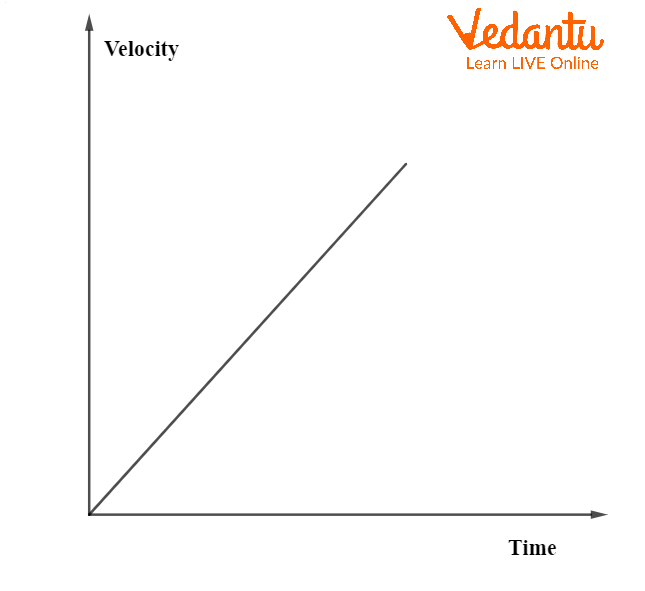Velocity v/s Time Graph for Uniform Acceleration

1. When the acceleration is uniform and the velocity is constantly decreasing, the graph obtained is a straight line with a negative slope. This negative acceleration is known as retardation.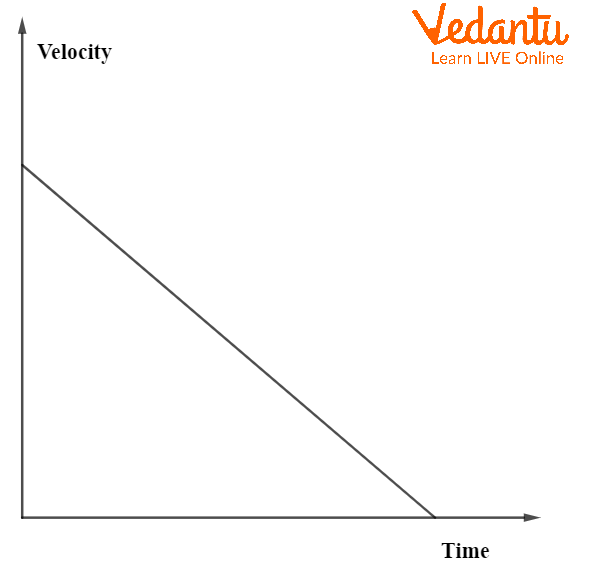Velocity v/s Time Graph for Uniform Retardation

1. When the acceleration is not uniform, e.g., if we accelerate for some time, and then slow down for a while, then the slope of the velocity-time graph also varies with time. Such a graph is shown below.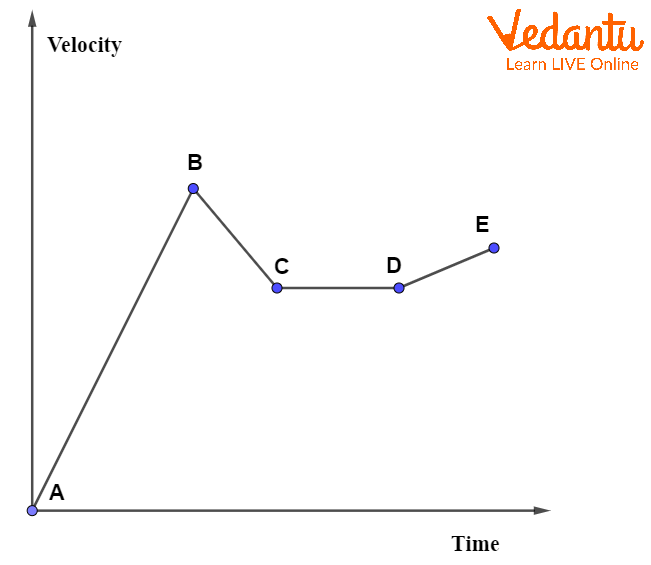Velocity v/s Time Graph for Non-uniform Acceleration

In the above graph, the velocity increases with a constant rate from A to B, then from B to C, the velocity decreases with a constant rate. From C to D, the velocity remains constant giving a straight horizontal line in the graph. Then, again from D to E, the velocity starts increasing with a constant rate. This is an illustration of non-uniform acceleration.

## Acceleration and Displacement from Velocity-Time Graph

The slope of a velocity time graph gives the acceleration of the motion. It can be determined by dividing the change in velocity by change in time as shown below.

Consider the following graph.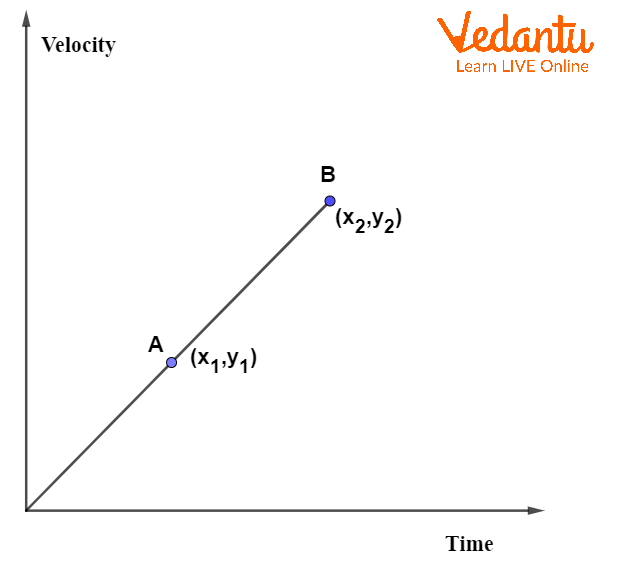Linear Velocity v/s Time Graph

The slope of displacement time graph is determined as $\dfrac{{{y}_{2}}-{{y}_{1}}}{{{x}_{2}}-{{x}_{1}}}$. This will be the acceleration (a) of the above graph.

$a=\dfrac{{{y}_{2}}-{{y}_{1}}}{{{x}_{2}}-{{x}_{1}}}$

How to find displacement in a velocity-time graph? The area under the velocity-time curve gives the displacement of the motion. It can be determined by multiplying the change in velocity with change in time. For example, we consider the above graph. The velocity change between points A and B will be ${{y}_{2}}-{{y}_{1}}$. The change in time is ${{x}_{2}}-{{x}_{1}}$. Then, the displacement between points A and B will be equal to $\left({{y}_{2}}-{{y}_{1}}\right) \times \left( {{x}_{2}}-{{x}_{1}} \right)$.

## Displacement-Acceleration Graph

Displacement-acceleration graph provides information about the variation in acceleration (and hence, force) with change in displacement. This graph basically helps find the value of force as a function of position. The acceleration-displacement graph of a particle in SHM shown below.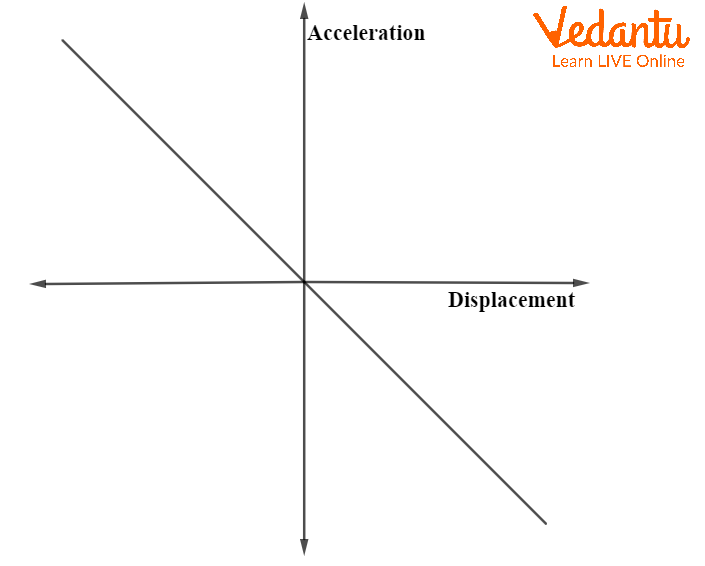Acceleration v/s Displacement Graph

## Conclusion

Motion, refers to the change of position with change in time. The shortest distance between the initial and final position of any object is termed as ‘displacement’. Velocity is defined as the displacement per unit time. Acceleration is defined as the rate of change of velocity. All three quantities, displacement, velocity and acceleration are vector quantities and can be negative, positive or zero under certain circumstances. The slope of the displacement time graph gives the velocity of the object in motion.

The slope of the displacement time graph gives the velocity of the object in motion. It can be determined by dividing the change in displacement by change in time. The slope of the velocity time graph gives the acceleration of the object in motion. It can be determined by dividing the change in velocity by change in time. The displacement can be determined from a velocity-time graph by multiplying the change in velocity with change in time.

Last updated date: 26th Sep 2023
Total views: 2.7k
Views today: 0.02k

## FAQs on Displacement, Velocity and Acceleration Time Graphs for JEE

1. Give an example where speed is different from velocity.

Let’s consider the following example. A man starts walking from the initial position towards the west direction. After 400 m, he turned right and walked 200 m towards the south. If he covered the distance in 20 minutes, then find the average speed and velocity in m/min. The speed will be determined by dividing the total distance by total time taken. The total distance is 600 m.

So, the speed will be $\dfrac{600}{20}=30m/\min$.

The velocity will be determined by dividing the displacement by total time taken. The displacement will be the short distance between initial and final position. The motion of the man is described in the below diagram.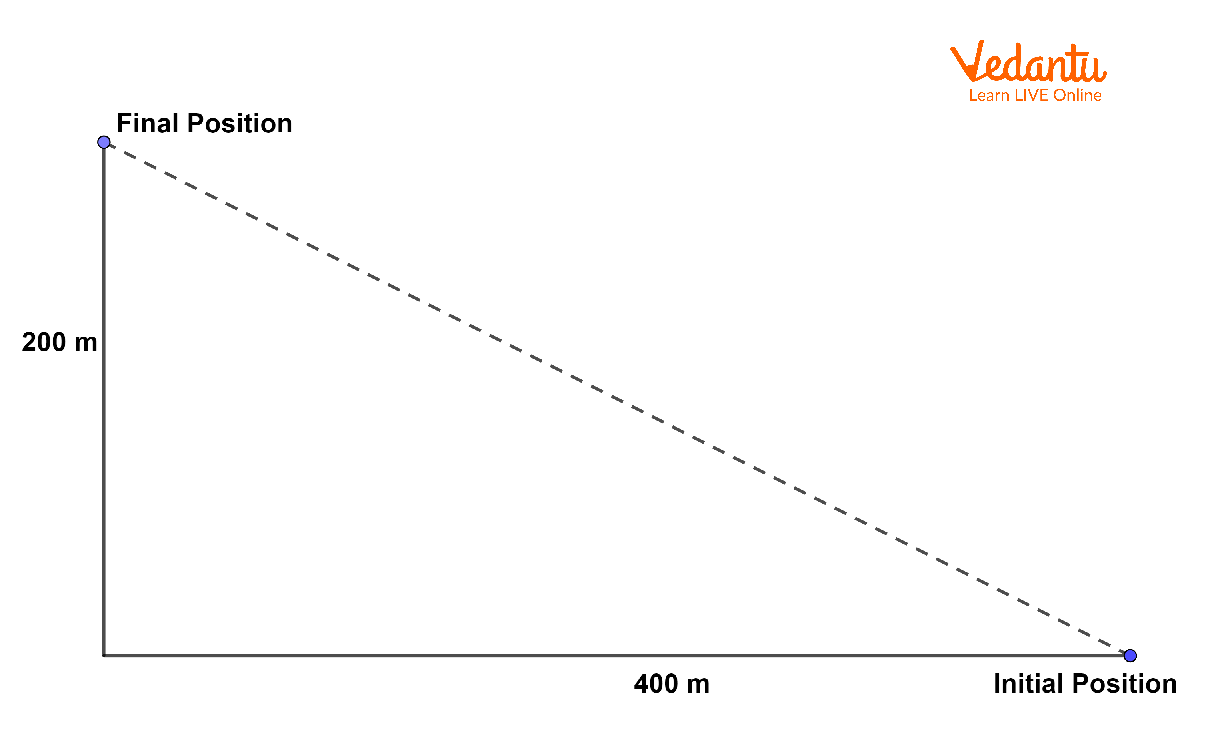Motion of the Man

The shortest distance is shown by the dashed line, which will be equal to

$\sqrt{{{\left( 400 \right)}^{2}}+{{\left( 200 \right)}^{2}}}\approx 447.21m.$

Therefore, the velocity will be equal to $\dfrac{447.21}{20}=22.36m/\min .$

We can see that the velocity is different from the speed.

2. Is it possible for an object to be in motion even if the acceleration is zero?

Yes, at any instant, if acceleration of an object is zero, it is still possible that the object could be in motion. The reason is that zero or no acceleration means that there is no change in velocity of the object. This means that the object could be moving with a constant velocity. For example, let us consider a car that was at rest. Then, some force was applied to start the car and after attaining a certain velocity, now the car is moving with a constant velocity. So, in this case, even though the car is moving, the acceleration is zero.